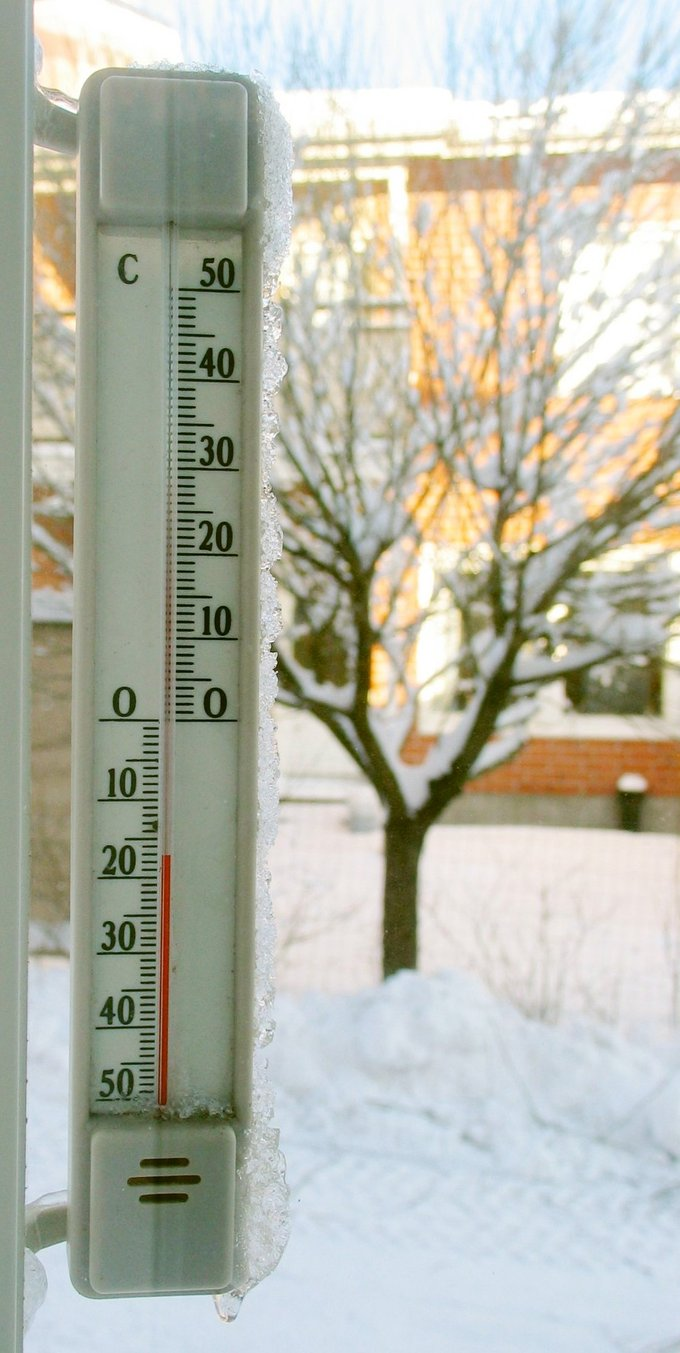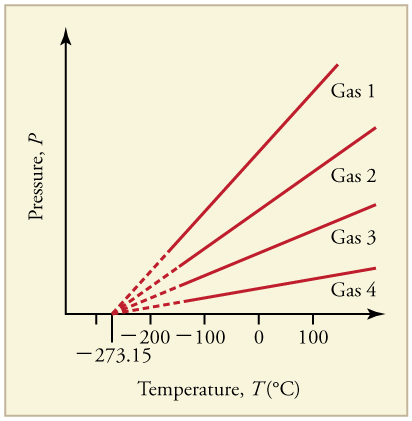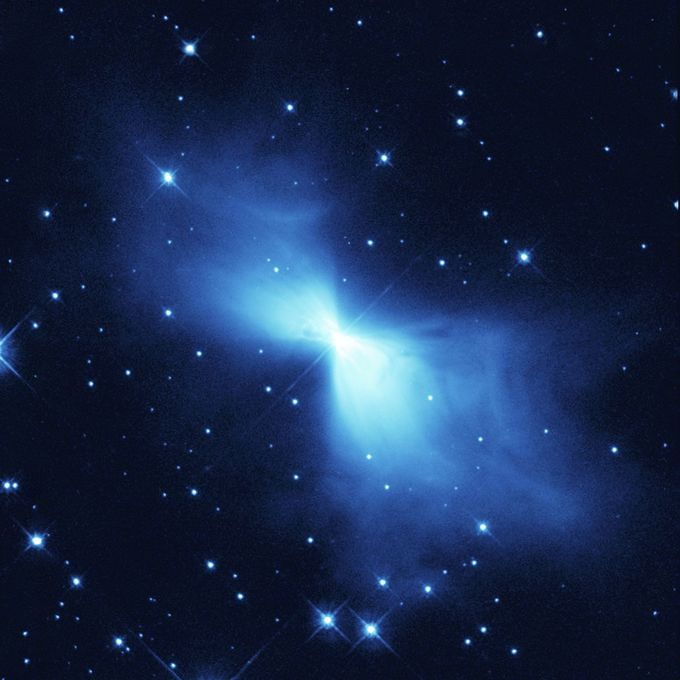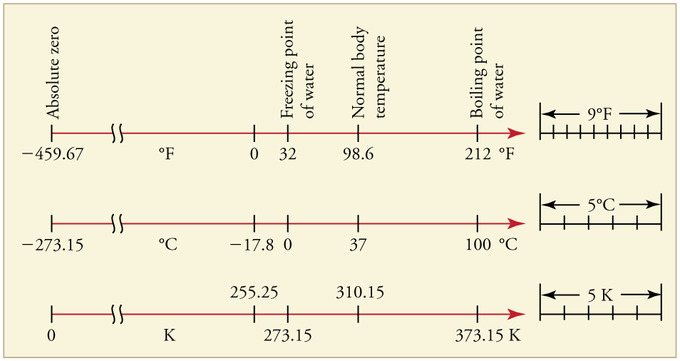## Celsius Scale

Celsius, or centigrade, is a scale and unit of measurement for temperature. It is one of the most commonly used temperature units.

### Learning Objectives

Explain how the Celsius scale is defined

### Key Takeaways

#### Key Points

• The degree Celsius (°C) can refer to a specific temperature on the Celsius scale as well as a unit to indicate a temperature interval, a difference between two temperatures or an uncertainty.
• The Celsius scale is currently defined by two different temperatures: absolute zero and the triple point of Vienna Standard Mean Ocean Water (VSMOW; specially purified water).
• Based on this, the relationship between Celsius and Kelvin is as follows: $\text{T}_{\text{Celsius}} = \text{T}_{\text{Kelvin}} -273.15$.

#### Key Terms

• kelvin: in the International System of Units, the base unit of thermodynamic temperature; 1/273.16 of the thermodynamic temperature of the triple point of water; symbolized as K
• absolute zero: The coldest possible temperature: zero on the Kelvin scale and approximately -273.15°C and -459.67°F. The total absence of heat; the temperature at which motion of all molecules would cease.
• standard atmosphere: an international reference pressure defined as 101.325 kPa and formerly used as a unit of pressure

Celsius, also known as centigrade, is a scale to measure temperature. The unit of measurement is the degree Celsius (°C). It is one of the most commonly used temperature units in the world. The unit system is named after the Swedish astronomer Anders Celsius (1701-1744), who developed a similar temperature scale.

Temperature Scales: A brief introduction to temperature and temperature scales for students studying thermal physics or thermodynamics.Thermometer: A thermometer calibrated in degrees Celsius

From 1743 until 1954, 0°C was defined as the freezing point of water, and 100°C was defined as the boiling point of water, both at a pressure of one standard atmosphere, with mercury as the working material. Although these defining correlations are commonly taught in schools today, by international agreement the unit “degree Celsius” and the Celsius scale are currently defined by two different temperatures: absolute zero and the triple point of Vienna Standard Mean Ocean Water (VSMOW; specially purified water). This definition also precisely relates the Celsius scale to the Kelvin scale, which defines the SI base unit of thermodynamic temperature and which uses the symbol K. Absolute zero, the lowest temperature possible (the temperature at which matter reaches minimum entropy), is defined as being precisely 0K and -273.15°C. The temperature of the triple point of water is defined as precisely 273.16K and 0.01°C. Based on this, the relationship between degree Celsius and Kelvin is as follows:Phase Diagram of Water: In this typical phase diagram of water, the green lines mark the freezing point, and the blue line marks the boiling point, showing how they vary with pressure. The dotted line illustrates the anomalous behavior of water. Note that water changes states based on the pressure and temperature.

$\text{T}_{\text{Celsius}} = \text{T}_{\text{Kelvin}} -273.15$.

Besides expressing specific temperatures along its scale (e.g., “Gallium melts at 29.7646°C” and “The temperature outside is 23 degrees Celsius”), the degree Celsius is also suitable for expressing temperature intervals — differences between temperatures, or their uncertainties (e.g. “The output of the heat exchanger is hotter by 40 degrees Celsius” and “Our standard uncertainty is ±3°C”). Because of this dual usage, one must not rely upon the unit name or its symbol to denote that a quantity is a temperature interval; it must be clear through context or explicit statement that the quantity is an interval.

## Farenheit Scale

In the Fahrenheit scale, the freezing of water is defined at 32 degrees, while the boiling point of water is defined to be 212 degrees.

### Learning Objectives

Explain how the Fahrenheit scale is defined and convert between it and Celsius

### Key Takeaways

#### Key Points

• The Fahrenheit system puts the boiling and freezing points of water exactly 180 degrees apart. Therefore, a degree on the Fahrenheit scale is 1⁄180 of the interval between the freezing point and the boiling point.
• To convert °F to °C, you can use the following formula: $\text{T}_{\text{Celsius}} = \frac{5}{9}(\text{T}_{\text{Fahrenheit}}-32)$. The Fahrenheit and Celsius scales intersect at −40°.
• The Fahrenheit scale was replaced by the Celsius scale in most countries during the mid to late 20th century. Fahrenheit remains the official scale of the United States, Cayman Islands, Palau, Bahamas and Belize.

#### Key Terms

• brine: a solution of salt (usually sodium chloride) in water
• frigorific mixture: A mixture of two or more chemicals that reaches an equilibrium temperature independent of the temperature of any of its constituent chemicals. The temperature is also relatively independent of the quantities of mixtures as long as a significant amount of each original chemical is present in its pure form

The Fahrenheit scale measures temperature. It is based on a scale proposed in 1724 by physicist Daniel Gabriel Fahrenheit (1686-1736). The unit of this scale is the degree Fahrenheit (°F). On this scale, water’s freezing point is defined to be 32 degrees, while water’s boiling point is defined to be 212 degrees.

Historically, the zero point of the Fahrenheit scale was determined by evaluating a thermometer placed in brine. Fahrenheit himself used a mixture of ice, water, and ammonium chloride (a salt) at a 1:1:1 ratio. This is a frigorific mixture, which stabilizes its temperature automatically; the stable temperature of this mixture was defined as 0 °F (-17.78 °C). The second determining point, 32 degrees, was a mixture of just ice and water at a 1:1 ratio. The third determining point, 96 degrees, was approximately the temperature of the human body, then called “blood-heat. ”

The Fahrenheit system puts the boiling and freezing points of water exactly 180 degrees apart. Therefore, a degree on the Fahrenheit scale is 1/180 of the interval between the freezing point and the boiling point. On the Celsius scale, the freezing and boiling points of water are 100 degrees apart. A temperature interval of 1 °F is equal to an interval of 5/9 degrees Celsius (°C). To convert °F to °C, you can use the following formula:

$\text{T}_{\text{Celsius}} = \frac{5}{9}(\text{T}_{\text{Fahrenheit}}-32)$

The Fahrenheit and Celsius scales intersect at -40° (-40 °F and -40 °C represent the same temperature). Absolute zero (-273.15 °C, or 0K) is defined as -459.67 °F.

The Fahrenheit scale was replaced by the Celsius scale in most countries in the mid- to late-20th century, though Canada retains it as a supplementary scale that can be used alongside the Celsius scale. The Fahrenheit scale remains the official scale of the United States, the Cayman Islands, Palau, the Bahamas, and Belize.Fig 2: Comparison of Celsius vs Fahrenheit scales.

Temperature Scales: A brief introduction to temperature and temperature scales for students studying thermal physics or thermodynamics.

## Absolute Zero

Absolute zero is the coldest possible temperature; formally, it is the temperature at which entropy reaches its minimum value.

### Learning Objectives

Explain why absolute zero is a natural choice as the null point for a temperature unit system

### Key Takeaways

#### Key Points

• Absolute zero is universal in the sense that all matter is in ground state at this temperature. Therefore, it is a natural choice as the null point for a temperature unit system.
• K system at absolute zero still possesses quantum mechanical zero-point energy, the energy of its ground state. However, in the interpretation of classical thermodynamics, kinetic energy can be zero, and the thermal energy of matter vanishes.
• The lowest temperature that has been achieved in the laboratory is in the 100 pK range, where pK (pico- Kelvin ) is equivalent to 10-12 K. The lowest natural temperature ever recorded is approximately 1K, observed in the rapid expansion of gases leaving the Boomerang Nebula.

#### Key Terms

• entropy: A measure of how evenly energy (or some analogous property) is distributed in a system.
• thermodynamics: a branch of natural science concerned with heat and its relation to energy and work

Absolute zerois the coldest possible temperature. Formally, it is the temperature at which entropy reaches its minimum value. More simply put, absolute zero refers to a state in which all the energy of a system is extracted (by definition, the lowest energy state the system can have). Absolute zero is universal in the sense that all matteris in ground state at this temperature. Therefore, it is a natural choice as the null point for a temperature unit system.Graph of Pressure Versus Temperature: Graph of pressure versus temperature for various gases kept at a constant volume. Note that all of the graphs extrapolate to zero pressure at the same temperature

To be precise, a system at absolute zero still possesses quantum mechanical zero-point energy, the energy of its ground state. The uncertainty principle states that the position of a particle cannot be determined with absolute precision; therefore a particle is in motion even if it is at absolute zero, and a ground state still carries a minimal amount of kinetic energy. However, in the interpretation of classical thermodynamics, kinetic energy can be zero, and the thermal energy of matter vanishes.

The zero point of a thermodynamic temperature scale, such as the Kelvin scale, is set at absolute zero. By international agreement, absolute zero is defined as 0K on the Kelvin scale and as -273.15° on the Celsius scale (equivalent to -459.67° on the Fahrenheit scale). Scientists have brought systems to temperatures very close to absolute zero, at which point matter exhibits quantum effects such as superconductivity and superfluidity. The lowest temperature that has been achieved in the laboratory is in the 100 pK range, where pK (pico-Kelvin) is equivalent to 10-12 K. The lowest natural temperature ever recorded is approximately 1K, observed in the rapid expansion of gases leaving the Boomerang Nebula, shown below.Boomerang Nebula: The rapid expansion of gases resulting in the Boomerang Nebula causes the lowest observed temperature outside a laboratory.

Temperature Scales: A brief introduction to temperature and temperature scales for students studying thermal physics or thermodynamics.

## Kelvin Scale

The kelvin is a unit of measurement for temperature; the null point of the Kelvin scale is absolute zero, the lowest possible temperature.

### Learning Objectives

Explain how the Kelvin scale is defined

### Key Takeaways

#### Key Points

• 0K ( absolute zero ) is universal because all thermal motions of all matter are maximally suppressed at this temperature. Absolute zero is therefore the natural choice as the null point of the Kelvin scale.
• The Kelvin scale is used extensively in scientific work because a number of physical quantities, such as the volume of an ideal gas, are directly related to absolute temperature.
• To convert kelvin to degree Celsius, we use the following formula:$\text{T}_{\text{Celsius}} = \text{T}_{\text{Kelvin}} -273.15$.

#### Key Terms

• absolute zero: The coldest possible temperature: zero on the Kelvin scale and approximately -273.15°C and -459.67°F. The total absence of heat; the temperature at which motion of all molecules would cease.
• Triple point: The unique temperature and pressure at which the solid, liquid and gas phases of a substance are all in equilibrium.
• ideal gas: A hypothetical gas whose molecules exhibit no interaction and undergo elastic collision with each other and with the walls of the container.

The kelvin is a unit of measurement for temperature. It is one of the seven base units in the International System of Units (SI) and is assigned the unit symbol K. The Kelvin scale is an absolute, thermodynamic temperature scale using absolute zero as its null point. In the classical description of thermodynamics, absolute zero is the temperature at which all thermal motion ceases.

The choice of absolute zero as null point for the Kelvin scale is logical. Different types of matter boil or freeze at different temperatures, but at 0K (absolute zero), all thermal motions of any matter are maximally suppressed. The Kelvin scale is used extensively in scientific work because a number of physical quantities, such as the volume of an ideal gas, are directly related to absolute temperature.

The Kelvin scale is named after Glasgow University engineer and physicist William Thomson, 1st Baron Kelvin (1824-1907), who wrote of the need for an “absolute thermometric scale. ” Unlike the degree Fahrenheit and the degree Celsius, the kelvin is not referred to or typeset as a degree. The kelvin is the primary unit of measurement in the physical sciences, but it is often used in conjunction with the degree Celsius, which has the same magnitude. The kelvin is defined as the fraction 1/273.16 of the thermodynamic temperature of the triple point of water (exactly 0.01°C, or 32.018°F). To convert kelvin to degrees Celsius, we use the following formula:

$\text{T}_{\text{Celsius}} = \text{T}_{\text{Kelvin}} -273.15$

Subtracting 273.16K from the temperature of the triple point of water, 0.01°C, makes absolute zero (0K) equivalent to -273.15°C and -460°F.Calculating URelationships Between the Temperature Scales: Relationships between the Fahrenheit, Celsius, and Kelvin temperature scales, rounded to the nearest degree. The relative sizes of the scales are also shown

Temperature Scales: A brief introduction to temperature and temperature scales for students studying thermal physics or thermodynamics.